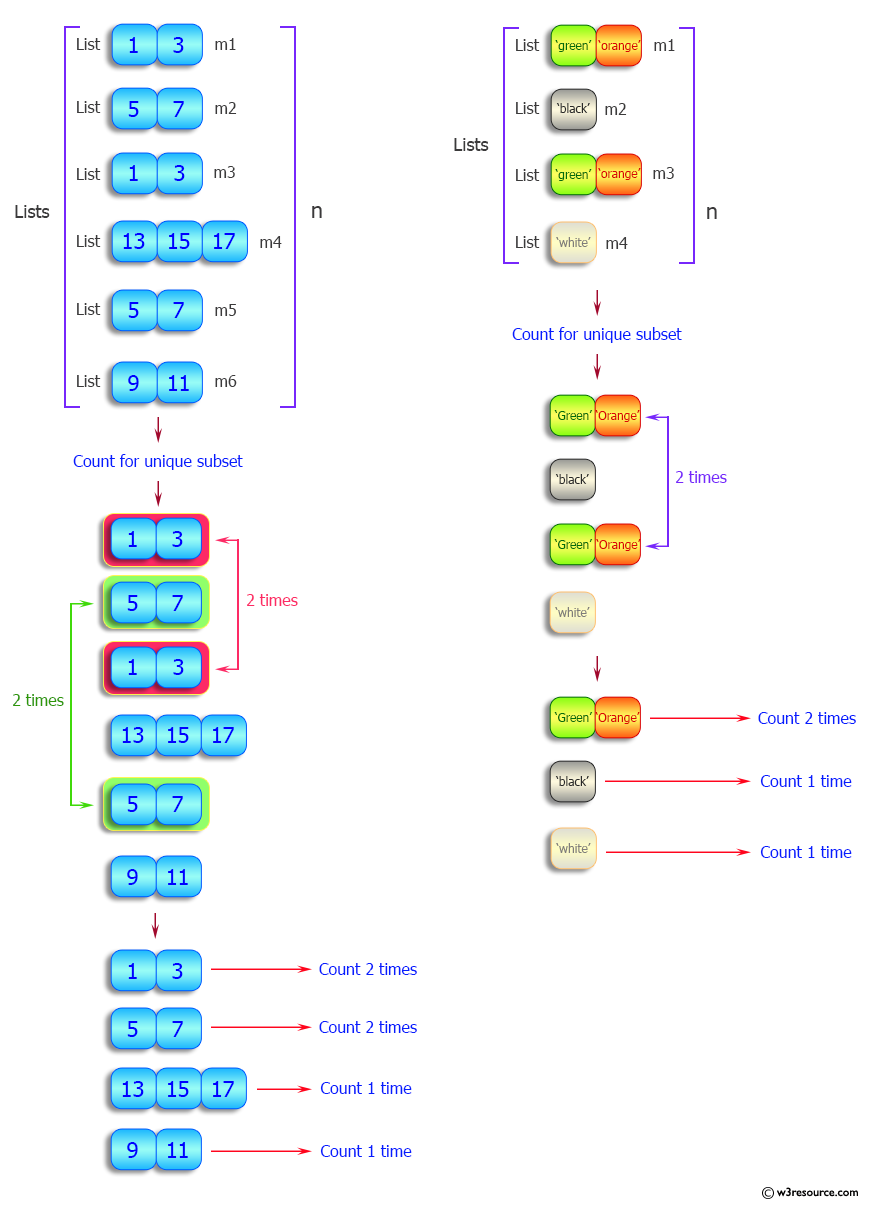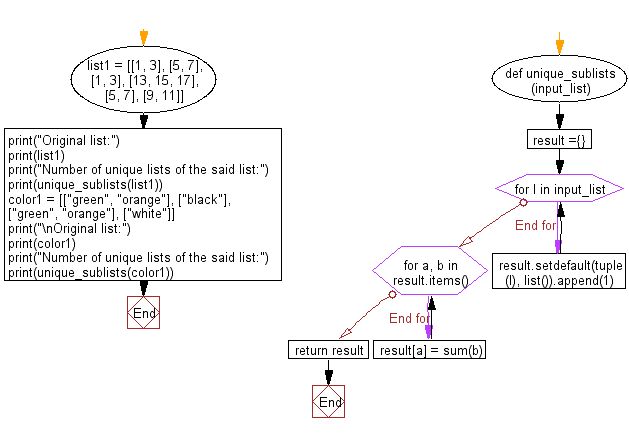﻿ Python: Count number of unique lists within list - w3resource# Python: Count number of unique lists within list

## Python List: Exercise - 94 with Solution

Write a Python program to count number of unique sublists within a given list.

Sample Solution:

Python Code:

``````def unique_sublists(input_list):
result ={}
for l in input_list:
result.setdefault(tuple(l), list()).append(1)
for a, b in result.items():
result[a] = sum(b)
return result

list1 = [[1, 3], [5, 7], [1, 3], [13, 15, 17], [5, 7], [9, 11]]
print("Original list:")
print(list1)
print("Number of unique lists of the said list:")
print(unique_sublists(list1))

color1 = [["green", "orange"], ["black"], ["green", "orange"], ["white"]]
print("\nOriginal list:")
print(color1)
print("Number of unique lists of the said list:")
print(unique_sublists(color1))
```
```

Sample Output:

```Original list:
[[1, 3], [5, 7], [1, 3], [13, 15, 17], [5, 7], [9, 11]]
Number of unique lists of the said list:
{(1, 3): 2, (5, 7): 2, (13, 15, 17): 1, (9, 11): 1}

Original list:
[['green', 'orange'], ['black'], ['green', 'orange'], ['white']]
Number of unique lists of the said list:
{('green', 'orange'): 2, ('black',): 1, ('white',): 1}
```

Pictorial Presentation:Flowchart:## Visualize Python code execution:

The following tool visualize what the computer is doing step-by-step as it executes the said program:

Python Code Editor:

Have another way to solve this solution? Contribute your code (and comments) through Disqus.

What is the difficulty level of this exercise?

Test your Python skills with w3resource's quiz

﻿

## Python: Tips of the Day

Floor Division:

When we speak of division we normally mean (/) float division operator, this will give a precise result in float format with decimals.

For a rounded integer result there is (//) floor division operator in Python. Floor division will only give integer results that are round numbers.

```print(1000 // 300)
print(1000 / 300)```

Output:

```3
3.3333333333333335```